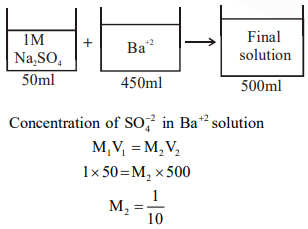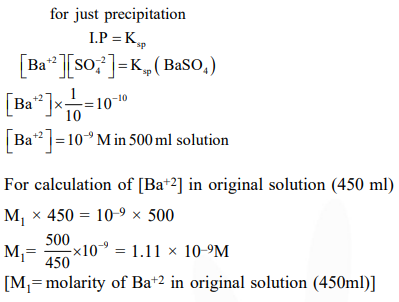# A aqueous solution contains an

Question:

A aqueous solution contains an unknown concentration of $\mathrm{Ba}^{2+}$. When $50 \mathrm{~mL}$ of a $1 \mathrm{M}$ solution of $\mathrm{Na}_{2} \mathrm{SO}_{4}$ is added, $\mathrm{BaSO}_{4}$ just begins to precipitate. The final volume is $500 \mathrm{~mL}$. The solubility product of $\mathrm{BaSO}_{4}$ is $1 \times 10^{-10}$. What is the original concentration of $\mathrm{Ba}^{2+}$ ?

1. $2 \times 10^{-9} \mathrm{M}$

2. $1.1 \times 10^{-9} \mathrm{M}$

3. $1.0 \times 10^{-10} \mathrm{M}$

4. $5 \times 10^{-9} \mathrm{MS}$

Correct Option: , 2

Solution: